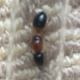Home > Grade 4 > The Largest Fraction That Is Less Than One Half

# The Largest Fraction That Is Less Than One Half

Directions: Using the digits 1 to 9 at most one time each, fill in the boxes to create the largest fraction possible that is less than 1/2 and has a single digit in both the numerator and denominator.

### Hint

How can you determine exactly how far a fraction is from 1/2? What must be true about the relationship between the numerator and denominator in this case?

### Answer

4/9.  Note if you remove the restriction of having a “single digit in both the numerator and denominator” there are other answers as Tim Clifford comments below.

Source: Dr. Brian Lack

## Multiplying Differences

Directions: Using the digits 1 to 9 at most one time each, fill in the …

### 2 comments

1.What about 486/973? That’s as close as I’ve got after a few minutes of playing. If you really want the answer to be 4/9, the question needs to be clearer.

•Great point Tim. Will make the change.